Homework Help Question & Answers

# 2. complex numbers a voltage v(t) is given: v(t)=v1(t)+v2(t)=1 V sin( omega t -45) – 2...

2. complex numbers
a voltage v(t) is given:
v(t)=v1(t)+v2(t)=1 V sin( omega t -45) – 2 V cos(omega t-60)

a)convert the voltages to phasors and sketch a phasor diagram of V1,V2 and V
b)reduce the expression of v(t) to the form v(t)=Vm cos(omega*t + fi(its angle))

do u see normaly 3 exercise _?

Problem 4. laplace transform of discontinuous function for the function illustrated a) sketch f'(t) b)find la place transform of f'(t)

#### Homework Answers

Answer #1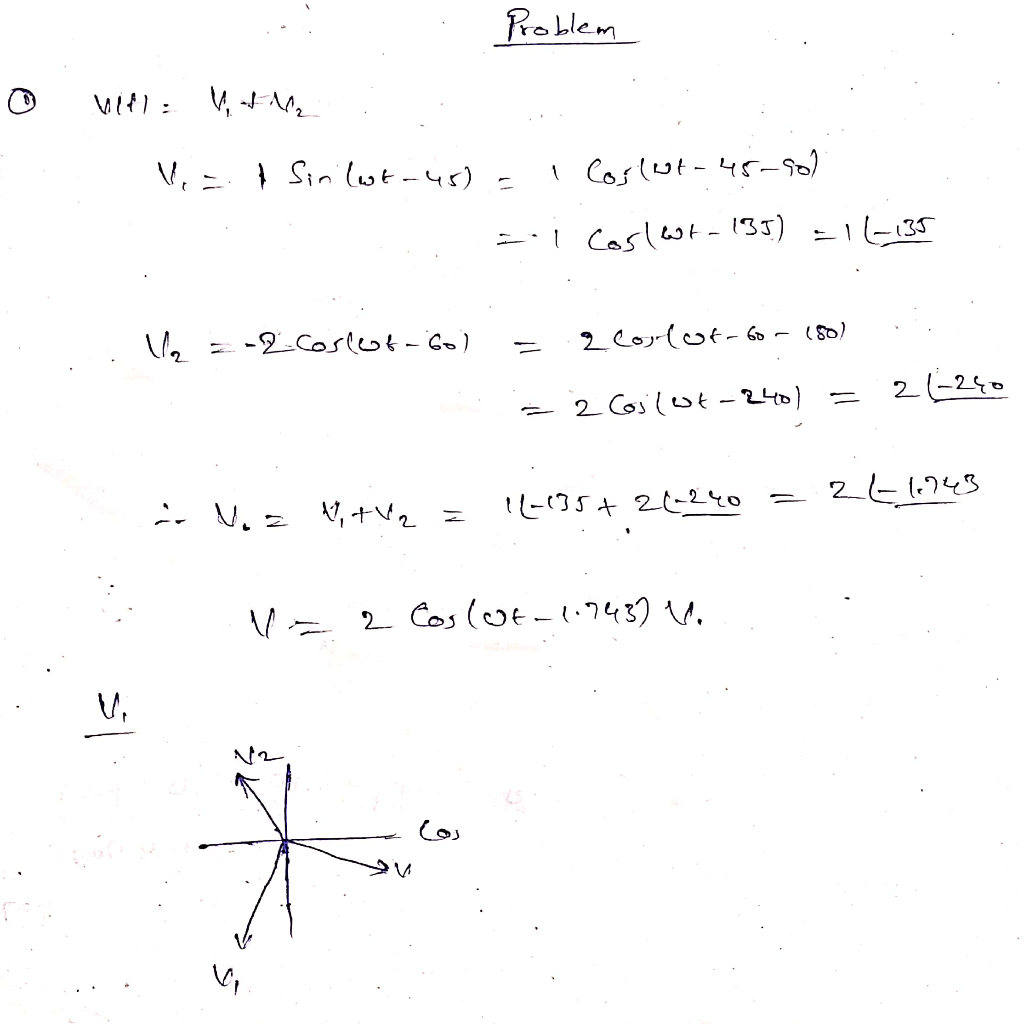Know the answer?
Your Answer:

#### Post as a guest

Your Name:

What's your source?

#### Earn Coin

Coins can be redeemed for fabulous gifts.

Not the answer you're looking for? Ask your own homework help question. Our experts will answer your question WITHIN MINUTES for Free.
Similar Homework Help Questions
• ### 300) V and v2 (t) 20 cos(wt 45°) V, find v(t) v1 (t)2(t) If v1(t) =...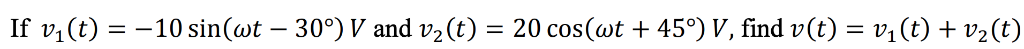300) V and v2 (t) 20 cos(wt 45°) V, find v(t) v1 (t)2(t) If v1(t) = -10 sin(wt _ -

• ### 1. (i) In a circuit, the voltage is given by: Vs (t) 12 cos(50t10) V (a)...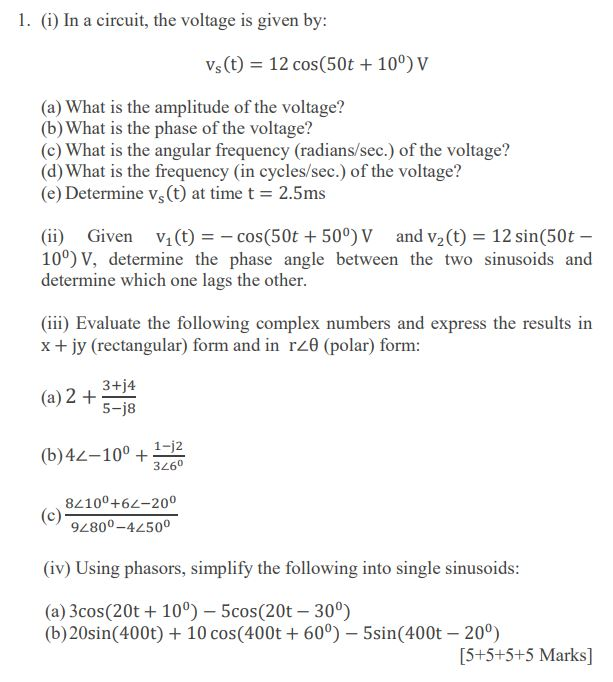1. (i) In a circuit, the voltage is given by: Vs (t) 12 cos(50t10) V (a) What is the amplitude of the voltage? (b) What is the phase of the voltage? (c) What is the angular frequency (radians/sec.) of the voltage? (d) What is the frequency (in cycles/sec.) of the voltage? (e) Determine vs (t) at time t 2.5ms (ii) Given v1(t)cos(50t 50°) V and v2(t) 12 sin(50t 10°) V, determine the phase angle between the two sinusoids and determine...

• ### USE DEFINITION 1 TO DETERMINE THE LAPLACE TRANSFORM OF THE FOLLOWING FUNCTION. f(t)= e sin(t) Laplace...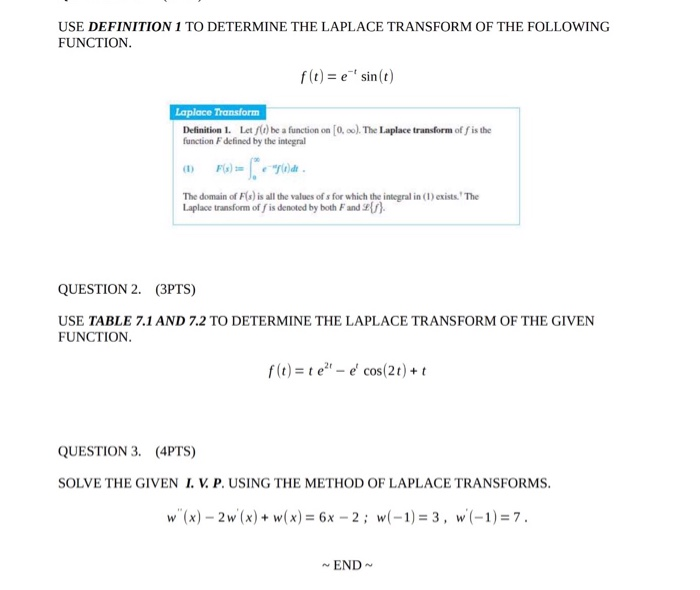USE DEFINITION 1 TO DETERMINE THE LAPLACE TRANSFORM OF THE FOLLOWING FUNCTION. f(t)= e sin(t) Laplace Transform Definition 1. Let f(t)be a function on [0,00). The Laplace transform of f is the function defined by the integral The domain of F(s) is all the values of " for which the integral in (1) exists.' The Laplace transform of fis denoted by both and \${/}. QUESTION 2. (3PTS) USE TABLE 7.1 AND 7.2 TO DETERMINE THE LAPLACE TRANSFORM OF THE GIVEN...

• ### (a) Write the relationship between the instantaneous time voltage i(t) = 1, cos(wt+p) and its phasor...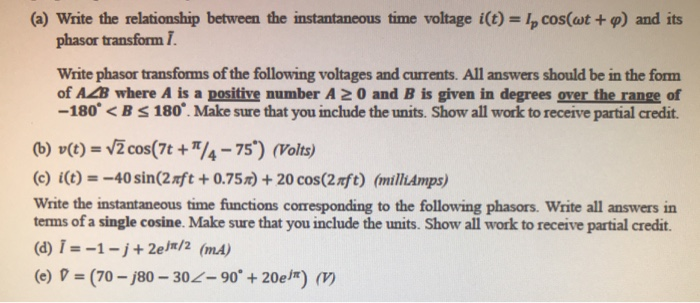(a) Write the relationship between the instantaneous time voltage i(t) = 1, cos(wt+p) and its phasor transform I. Write phasor transforms of the following voltages and currents. All answers should be in the form of AZB where A is a positive number A 20 and B is given in degrees over the range of -180° <B S 180°. Make sure that you include the units. Show all work to receive partial credit. (6) v(t) = VŽ cos(7t + 1/4 -...

• ### 8 H 2 Q iL Vs (t 22 1. vs (t) 2 V; this is a...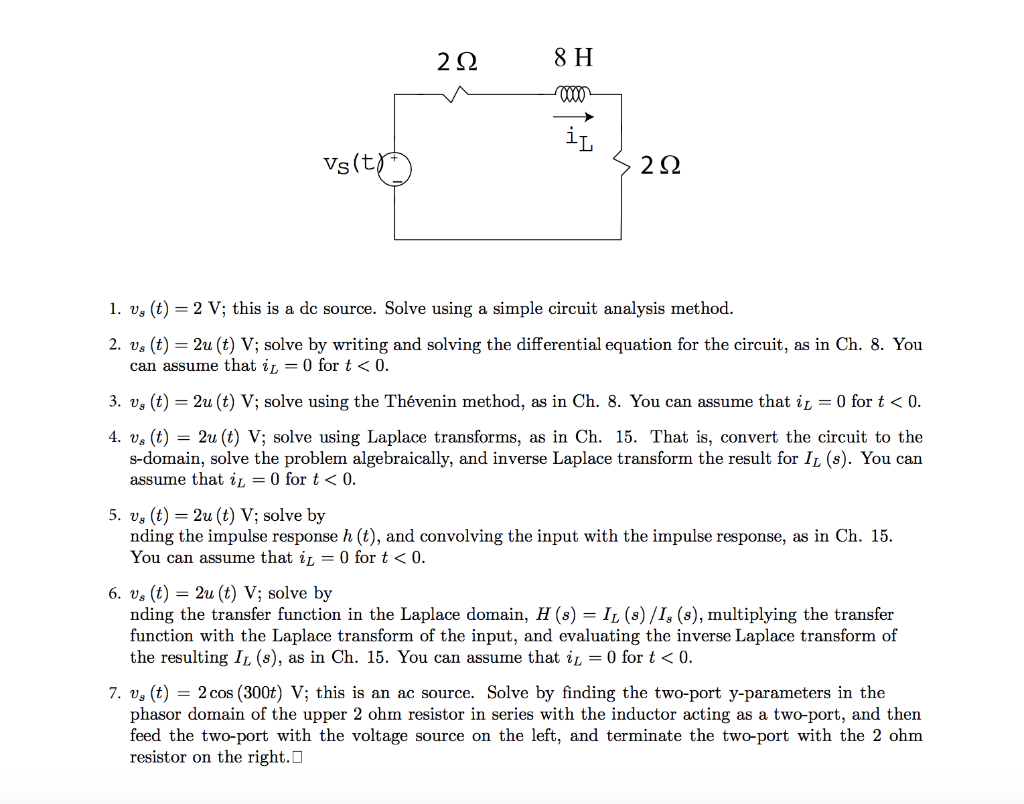8 H 2 Q iL Vs (t 22 1. vs (t) 2 V; this is a dc source. Solve using a simple circuit analysis method 2. Us (t) 2u (t) V; solve by writing and solving the differential equation for the circuit, as in Ch. 8. You = = 0 for t0. can assume that ir 2u (t) V; solve using the Thévenin method, as in Ch. 8. You can assume that i, = 0 for t< 0. 3. vg...

• ### 1. Determine the Laplace transform of the following signals e* .11(t) ; (b) g(t)=Icos(2) + sin(2t)j.u(1-3)...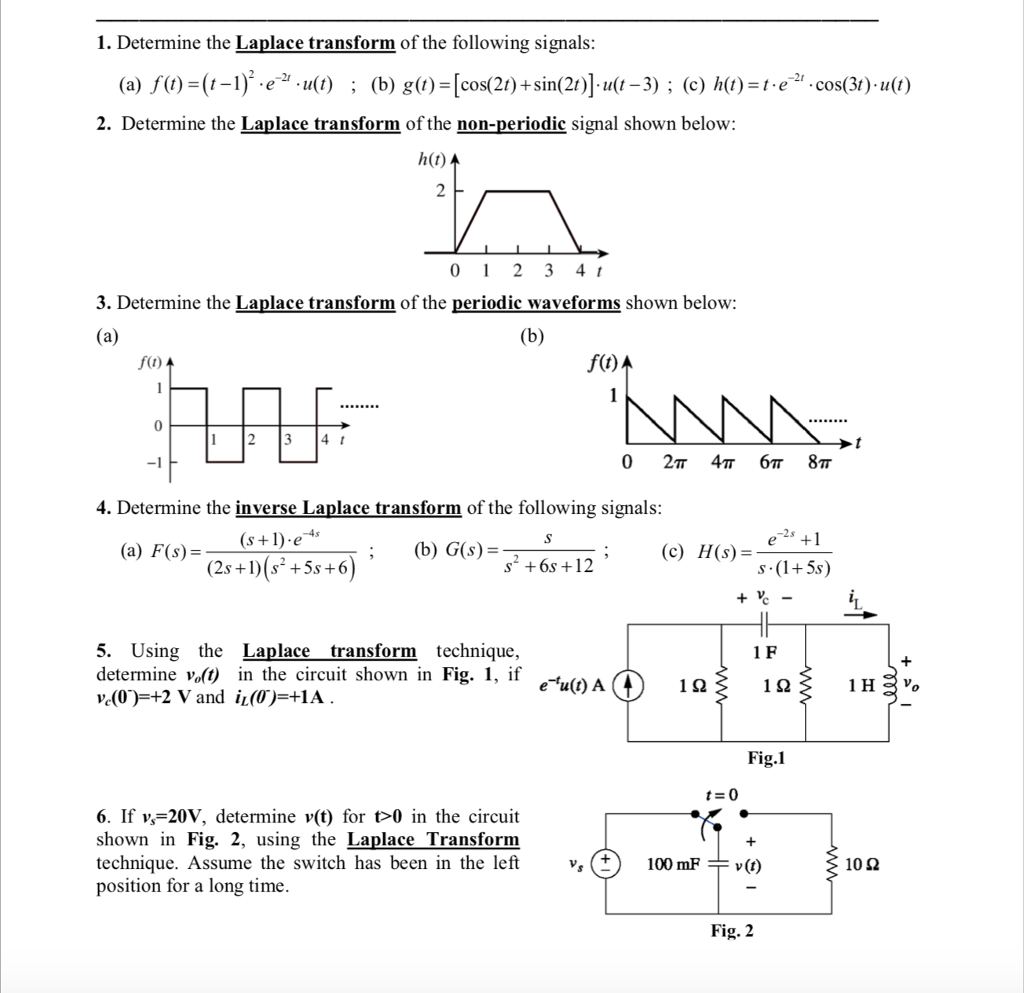1. Determine the Laplace transform of the following signals e* .11(t) ; (b) g(t)=Icos(2) + sin(2t)j.u(1-3) ; (c) h(t)-t-e-21. cos(30.11(1) 2. Determine the Laplace transform of the non-periodic signal shown below: h(t) 0 1 2 3 4 t 3. Determine the Laplace transform of the periodic waveforms shown below: fa) f(t) 0 2T 4T 6T 8T 4. Determine the inverse Laplace transform of the following signals 2s (b) G)6s+12 H(s) =s.(14%) (a) F(s)-De (c) (2s +1)(s1 +5s +6 5. Using...

• ### 4. Applications of Laplace transform Questions 1-4, find y(t) (1). y(t)=t+ſ v(7) sin(t – r)dt (2)....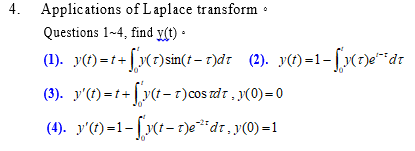4. Applications of Laplace transform Questions 1-4, find y(t) (1). y(t)=t+ſ v(7) sin(t – r)dt (2). y(t)=1-1 "(t)edt (3). y'(t)=t+ſ v(t = 1)cos edt , y(0)=0 (4). y'(t)=1-1 y(t-1) edt, y(0)=1

• ### Circuit Analysis with Phasors and Complex Impedances: Node-Voltage Analysis - Copy 2 of 4 - Part...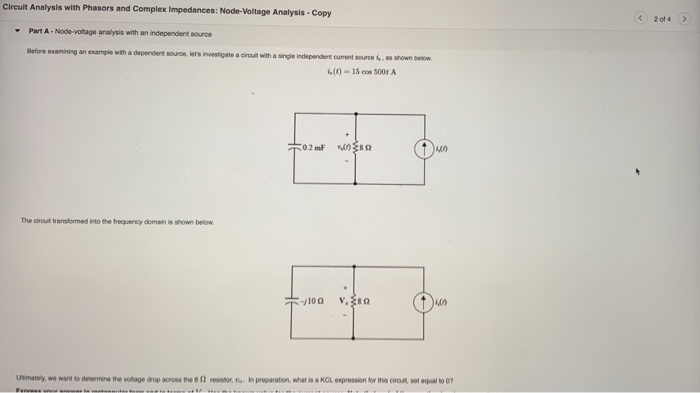Circuit Analysis with Phasors and Complex Impedances: Node-Voltage Analysis - Copy 2 of 4 - Part A - Node voltage analysis with an independent source Before examining an example with a dependent souron, was investigate a circuit with a single independent current source is shown below. (0) - 15 cod 500+ 50.2 m WS The circuit transformed into the frequency domain is shown below. -/100 V. Ultimately, we want to determine the voltage drop across the story in preparation, what...

• ### t for OSts 3 sketch the following orosts3 waveforms: (a) x(-t) 1. For the signal e) and (bl x(2-t) with appropriate labelling, (2+2-4 marks) 2. Recall that the energy of a continuous-time signal...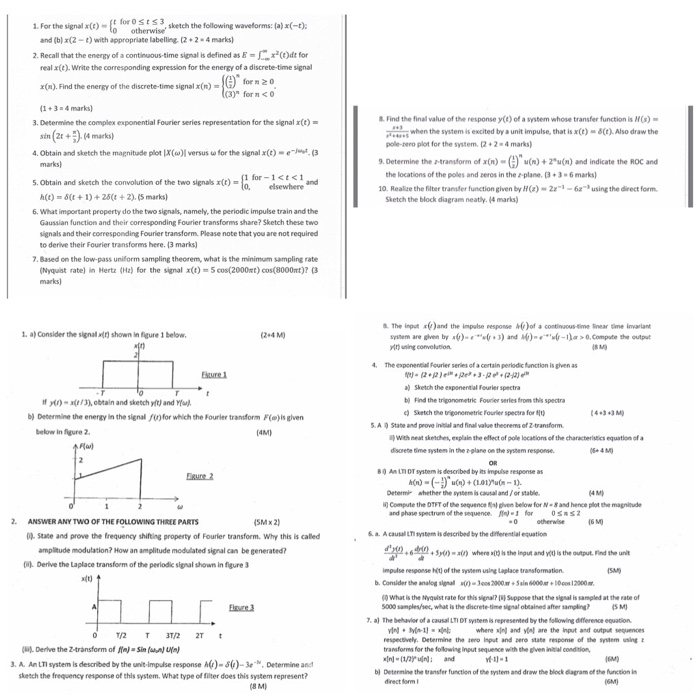t for OSts 3 sketch the following orosts3 waveforms: (a) x(-t) 1. For the signal e) and (bl x(2-t) with appropriate labelling, (2+2-4 marks) 2. Recall that the energy of a continuous-time signal is defined as E-匚x3(t)dt for real x(t). Write the corresponding expression for the energy of a discrete-time signal x(n). Find the energy of the discrete-time signal x(n)- 3) forn<0 1+3-4 marks & Find the final value of the response y(t) of a system whose transfer function is...

• ### 1. Why can the DSO only measure node voltages when the Function Generator is the power supply in ...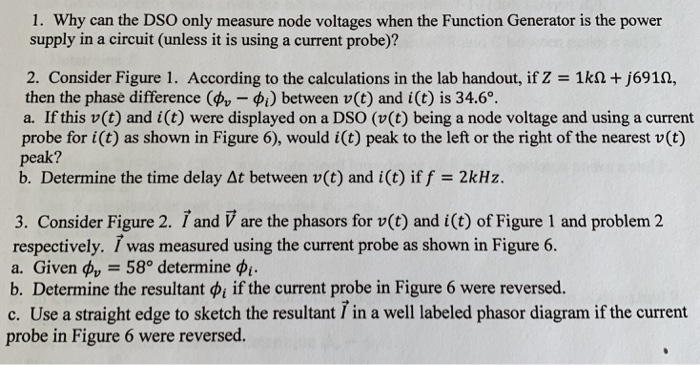1. Why can the DSO only measure node voltages when the Function Generator is the power supply in a circuit (unless it is using a current probe)? 2. Consider Figure 1. According to the calculations in the lab handout, if Z-1kΩ +/6914, then the phase difference (фи-фі) between u(t) and i (t) is 34.6". a. If this v(t) and i(t) were displayed on a DSO (v(t) being a node voltage and using a current probe for i(t) as shown in...